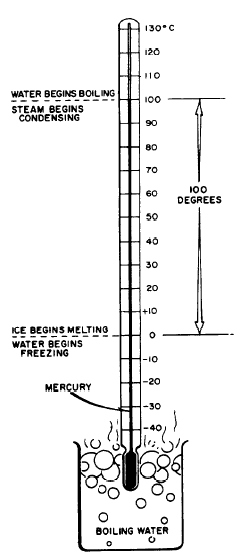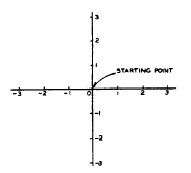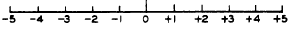Chapter 3 - SIGNED NUMBERSCustom SearchCHAPTER 3 SIGNED NUMBERS The positive numbers with which we have worked in previous chapters are not sufficient for every situation which may arise. For example, a negative number results in the operation of subtraction when the subtrahend is larger than the minuend. NEGATIVE NUMBERS When the subtrahend happens to be larger than the minuend, this fact is indicated by placing a minus sign in front of the difference, as in the following: 12 - 20 = -8 The difference, -8, is said to be NEGATIVE. A number preceded by a minus sign is a NEGATIVE NUMBER. The number -8 is read "minus eight." Such a number might arise when we speak of temperature changes. If -the temperature was 12 degrees yesterday and dropped 20 degrees today, the reading today would be 12 - 20, or -8 degrees. Numbers that show either a plus or minus sign are called SIGNED NUMBERS. An un- signed number is understood to be positive and is treated as though there were a plus sign preceding it. If it is desired to emphasize the fact that a number is positive, a plus sign is placed in front of the number, as in +5, which is read "plus five . �1 Therefore, either +5 or 5 indicates that the number 5 is positive. If a number is negative, a minus sign must appear in .front of it, as in -9. In dealing with signed numbers it should be emphasized that the plus and minus signs have two separate and distinct functions. They may indicate whether a number is positive or negative, or they may indicate the operation of addition or subtraction. When operating entirely with positive numbers, it is not necessary to be concerned with this distinction since plus or minus signs indicate only addition or subtraction. However, when negative numbers are also involved in a computation, it is important to distinguish between a sign of operation and the sign of a number. DIRECTION OF MEASUREMENT Signed numbers provide a convenient way of indicating opposite directions with a minimum of words. For example, an altitude of 20 ft above sea level could be designated as +20 ft. The same distance below sea level would then be designated as -20 ft. One of the most common devices utilizing signed numbers to indicate direction of measurement is the thermometer. Thermometer The Celsius (centigrade) thermometer shown in figure 3-l illustrates the use of positive and negative numbers to indicate direction of travel above and below 0. The 0 mark is the changeover point, at which the signs of the scale numbers change from - to +. When the thermometer is heated by the surrounding air or by a hot liquid in which it is placed, the mercury expands and travels up the tube. After the expanding mercury passes 0, the mark at which it comes to rest is read as a positive temperature. If the thermometer is allowed to cool, the mercury contracts. After passing 0 in its downward movement, any mark at which it comes to rest is read as a negative temperature. Rectangular Coordinate System As a matter of convenience, mathematicians have agreed to follow certain conventions as to the use of signed numbers in directional measurement. For example, in figure 3-2, a direction to the right along the horizontal line is positive, while the opposite direction (toward the left) is negative. On the vertical line, direction upward is positive, while direction downward is negative. A distance of -3 units along the horizontal line indicates a measurement of 3 units to the left of starting point 0. A distance of -3 units on the vertical line indicatesFigure 3-l.-Celsius (centigrade) temperature scale. a measurement of 3 units below the starting point. The two lines of the rectangular coordinate system which pass through the 0 position are the vertical axis and horizontal axis. Other vertical and horizontal lines may be included, forming a grid. When such a grid is used for the location of points and lines, the resulting "picture" containing points and lines is called a GRAPH.Figure 3-2. -Rectangular coordinate system. The Number Line Sometimes it is important to know the relative greatness (magnitude) of positive and negative numbers. To determine whether a particular number is greater or less than another number, think of all the numbers both positive and negative as being arranged along a horizontal line. (See fig. 3-3.)Figure 3-3.-Number line showing both positive and negative numbers.  Place zero at the middle of the line. Let the positive numbers extend from zero toward the right. Let the negative numbers extend from zero toward the left. With this arrangement, positive and negative numbers are so located that they progress from smaller to larger numbers as we move from left to right along the line. Any number that lies to the right of a given number is greater than the given number. A number that lies to the left of a given number is less than the given number. This arrangement shows that any negative number is smaller than any positive number.  The symbol for "greater than" is >. The symbol for "less than" is <. It is easy to distinguish between these symbols because the symbol used always opens toward the larger number. For example, "7 is greater than 4" can be written 7 > 4 and "-5 is less than -1" can be written -5 < -1. Absolute Value The ABSOLUTE VALUE of a number is its numerical value when the sign is dropped. The absolute value of either +5 or -5 is 5. Thus, two numbers that differ only in sign have the same absolute value. The symbol for absolute value consists of two vertical bars placed one on each side of the number, as in |-5| = 5. Consider also the following: |4 - 20|= 16 |+7| = |-7| = 7 The expression |-7| is read "absolute value of minus seven." When positive and negative numbers are used to indicate direction of measurement, we are concerned only with absolute value, if we wish to know only the distance covered. For example, in figure 3-2, if an object moves to the left from the starting point to the point indicated by -2, the actual distance covered is 2 units. We are concerned only with the fact that |-2| = 2, if our only interest is in the distance and not the direction.Integrated Publishing, Inc. - A (SDVOSB) Service Disabled Veteran Owned Small Business